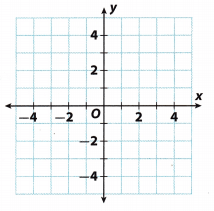# Texas Go Math Grade 8 Lesson 4.3 Answer Key Graphing Linear Nonproportional Relationships using Slope and y-Intercept

Refer to our Texas Go Math Grade 8 Answer Key Pdf to score good marks in the exams. Test yourself by practicing the problems from Texas Go Math Grade 8 Lesson 4.3 Answer Key Graphing Linear Nonproportional Relationships using Slope and y-Intercept.

## Texas Go Math Grade 8 Lesson 4.3 Answer Key Graphing Linear Nonproportional Relationships using Slope and y-Intercept

Essential Question
How can you graph a line using the slope and y-intercept?

Reflect

Question 1.
Draw Conclusions How can you use the slope of a line to predict the way the line will be slanted? Explain.
If the slope is positive, the graph will be slanted upwards and if the slope is negative, the graph will be slanted downwards.

Graph each equation.

Question 2.
y = $$\frac{1}{2}$$x + 1From the given equation y = $$\frac{1}{2}$$x + 1, we can see that y-intercept (‘b”) is 1. The first point is (0, b) → (0, 1).

To find the second point we can use the slope (“m”), thus m = $$\frac{1}{2}$$. Since the slope is positive, we are counting 1 unit up, and 2 units to the right, and our next point is (2, 2). Plot it in the graph: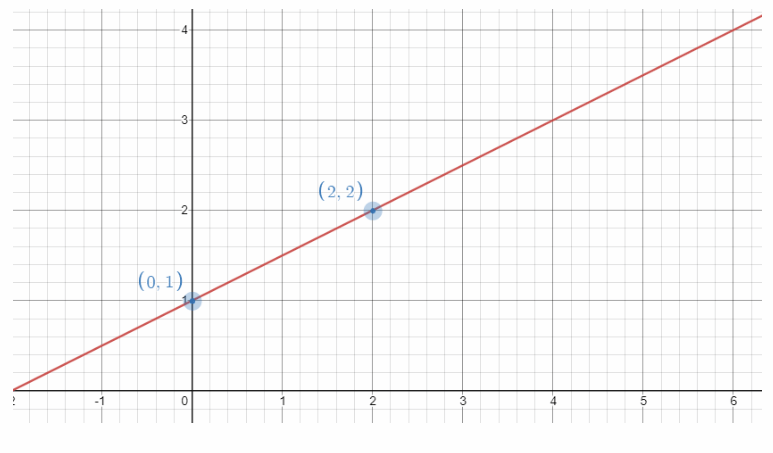Question 3.
y = -3x + 4y = -3x + 4
The y intercept is b = 4
Plot the point that contains the y-intercept:
(0, 4)
The slope is m = $$\frac{-3}{1}$$
Use the slope to find a second point.
From
(0, 4)
count $-3$ unit down and $1$ unit right. The new point is
(1, 1)
Draw a line through the points.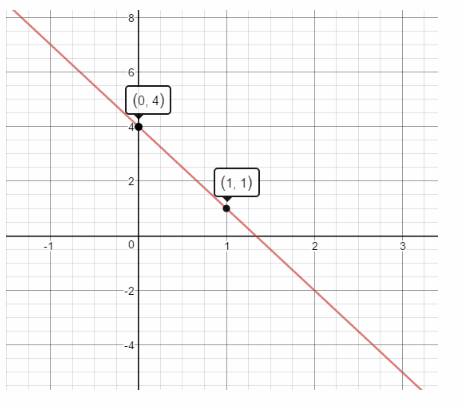Example 2.
Ken has a weekly goal for the number of calories he will burn by taking brisk walks. The equation y = -300x + 2400 represents the number of calories y Ken has left to burn after x hours of walking.
A. Graph the equation y = -300x + 2400.
Step 1
Write the slope as a fraction.
m = $$\frac{-300}{1}$$ = $$\frac{-600}{2}$$ = $$\frac{-900}{3}$$
Step 2
Plot the point for the y-intercept:
(0, 2400).
Step 3
Use the slope to locate a second point.From (0, 2400), count down 900 and right 3.
The new point is (3, 1500).
Step 4
Draw a line through the two points.

B. After how many hours of walking will Ken have 600 calories left to burn? After how many hours will he reach his weekly goal?
Step 1
Locate 600 calories on the y-axis. Read across and down to the x-axis.
Ken will have 600 calories left to burn after 6 hours.Step 2
Ken will reach his weekly goal when the number of calories left to burn is 0. Because every point on the x-axis has a y-value of 0, find the point where the line crosses the x-axis.
Ken will reach his goal after 8 hours of brisk walking.

What If? Ken decides to modify his exercise plans from Example 2 by slowing the speed at which he walks. The equation for the modified plan is y = -200x + 2400.

Question 4.
Graph the equation.
y = -200x + 2400.
Write the slope as a fraction
$$\frac{-200}{1}$$ = $$\frac{-400}{2}$$
The y intercept is b = 2400
Plot the point that contains the y-intercept:
(0, 2400)
The slope is m = $$\frac{-400}{2}$$
Use the slope to find a second point
From
(0, 2400)
count $400$ unit down and $2$ unit right. The new point is
(2, 2000)
Draw a line through the points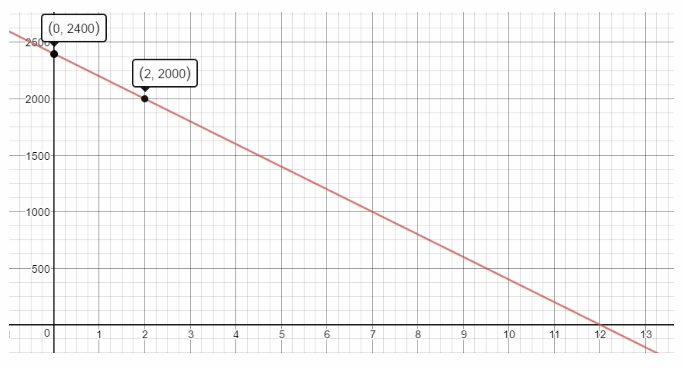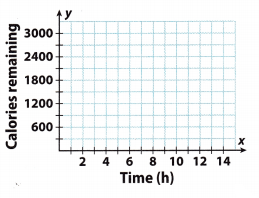Question 5.
How does the graph of the new equation compare with the graph in Example 2?
The y-intercept of the second graph ¡s the same with the y-intercept of the first graph: (0, 2400).
The slope (m) of the second graph (-200) is greater than the slope of the first graph (-300), therefore we can see
that Ken will reach his weekly goal earlier in the first graph compared with the second one. The x-intercept of the first graph is (8, 0), while the x-irtercept of the second graph is (12, 0).

Question 6.
Will Ken have to exercise more or less to meet his goal? Explain.
He will have to exercise more to meet his goal. He burns less calories per hour or the calories left to burn will decrease more slowly per hour.

Question 7.
Suppose Ken decides that instead of walking, he will jog, and jogging burns 600 calories per hour. How do you think this would change the graph?
The slope of the new graph will become -600, but the y intercept remains the same. The graph would be steeper and the line would intersect the x-axis when x = 4 hrs

Math Talk
Mathematical processes
What do the slope and the y-intercept of the line represent in this situation?

Graph each equation using the slope and the y-intercept. (Example 1)

Question 1.
y = $$\frac{1}{2}$$x – 3
slope = ____ y-intercept = ___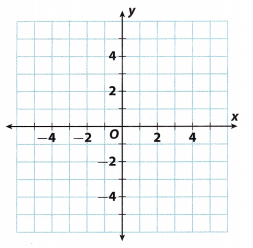y = $$\frac{1}{2}$$x – 3
The y intercept is b = – 3
Plot the point that contains the y-intercept:
(0, -3)
The slope is m = $$\frac{1}{2}$$
Use the slope to find a second point
From
(0, -3)
count $1$ unit up and $2$ unit right. The new point is
(2, -2)

Draw a line through the pointsQuestion 2.
y = -3x + 2
slope = ____ y-intercept = ___y = -3x + 2
The y intercept is b = 2
Plot the point that contains the y-intercept:
(0, 2)
The slope is m = $$\frac{-3}{1}$$
Use the slope to find a second point
From
(0, 2)
count $3$ unit down and $1$ unit right. The new point is
(1, -1)
Draw a line through the points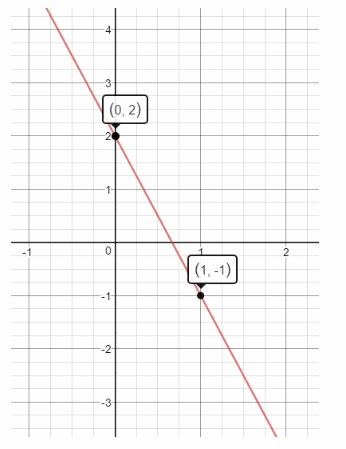Question 3.
A friend gives you two baseball cards for your birthday. Afterward, you begin collecting them. You buy the same number of cards once each week. The equation y = 4x + 2 describes the number of cards, y, you have after x weeks. (Example 2)
a. Find and interpret the slope and they-intercept of the line that represents this situation. Graph the equation y = 4x + 2.
Standard form of the given equation y = 4x + 2 is y = mx + b, so we already have values for the slope and
y-intercept (“b”). Thus, m = 4 and b = 2. The first point to plot in the graph is (0, b) → (0, 2).Using the slope we can find the second point but we have to know that m = 4 is the same as m = $$\frac{4}{1}$$ because we need to move the first point up/down for x units (number in the numerator) and several units right (number in the denominator).
So, from the first point we are counting 4 units up and 1 unit right and get the second point (16). Plot it in the
graph.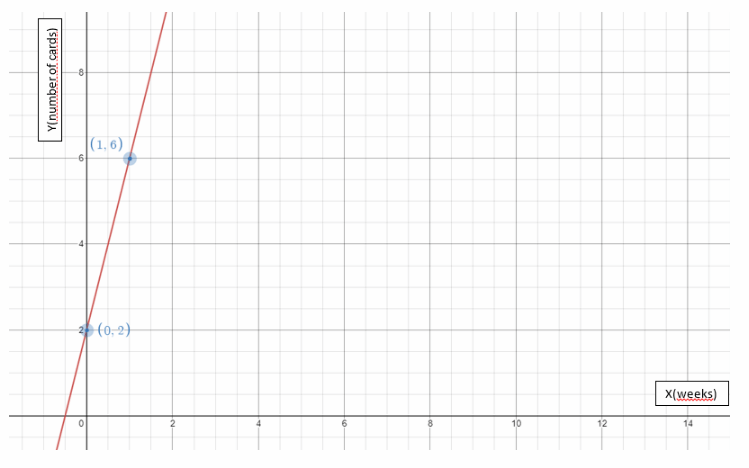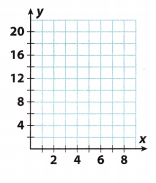b. Discuss which points on the line do not make sense in this situation. Then plot three more points on the line that do make sense.
To draw the points that will, be in the parameters of the function, I will include certain values for x in the given
equation (x indicates the number of weeks that have passed). The numbers I choose must be integers because the
values obtained for x y must also be integers. The y-axis indicates the number of cards collected and in reality
can not be counted as fractions.

So, for the x will take 2 (two weeks…), 3, and 5.
Thus:
y = 4x + 2
y = 4 . 2 + 2
y = 8 + 2
y = 10
(2, 10)
y = 4 . 3 + 2
y = 12 + 2
y = 14
(3, 14)
y = 4 . 5 + 2
y = 20 + 2
y = 22
(5, 22)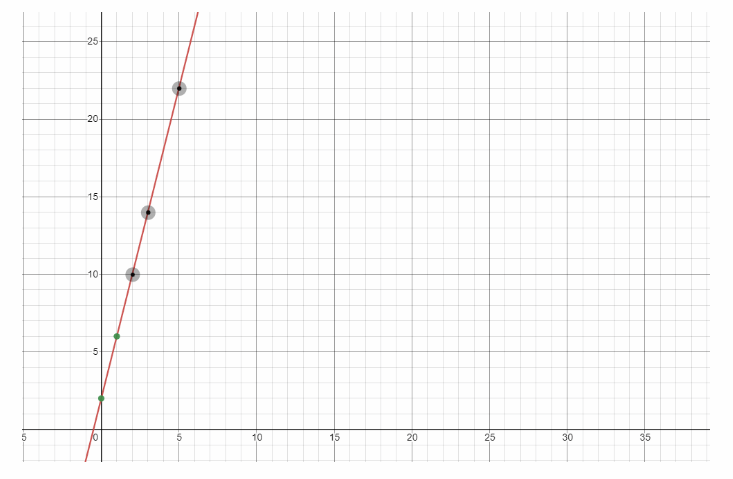Any point chosen in fractions between integers on the x-axis makes no sense because, in reality, it does not correspond to the parameters.

Essential Question Check-In

Question 4.
Why might someone choose to use the y-intercept and the slope to graph a line?
When the relationship is given ¡n the form y = mx b, the y-intercept (b) and the slope (m) are easily accessible and easily calculable. Therefore, it is a good practice to use them to graph the line.

Question 5.
Science A spring stretches in relation to the weight hanging from it according to the equation y = 0.75x + 0.25 where x is the weight in pounds and y is the length of the spring in inches.
a. Graph the equation.
y = 0.75x + 0.25
Slope (m) = 0.75
and
y-intercept = 0.25

Plot the point that contains the y-intercept:
(0, 0.25)
The slope is m = $$\frac{0.75}{1}$$
Use the slope to find a second point.
From
(0, 0.25)
count $0.75$ unit up and $1$ unit right. The new point is
(1, 1)
Draw a line through the pointsb. Interpret the slope and the y-intercept of the line.
Slope represents the increase in the length of spring in inches for each increase of pound of weight. y intercept represents the un-stretched length of the spring when there is no weight attached.

c. How long will the spring be if a 2-pound weight is hung on it? Will the length double if you double the weight? Explain
When there is a 2 pound weight hung, the length of the spring would be 1.75 inches.
No, when there is a 4 pound weight hung, the length of the spring would be 3.25 inches and not 3.5 inches.

Look for a Pattern Identify the coordinates of four points on the line with each given slope and y-intercept.

Question 6.
slope = 5, y-intercept = -1
Slope = 5
y – intercept = -1

Plot the point that contains the y-intercept:
(0, -1)

The slope is m = $$\frac{5}{1}$$
Use the slope to find a second point
From
(0, -1)
count $5$ unit up and $1$ unit right. The new point is
(1, 4)
Follow the same procedure to find the remaining three points.
(2, 9)
(3, 14)

Question 7.
slope = -1, y-intercept = 8
To determine the coordinates of four points on the line given the slope and y-intercept, Let us use the slope-
intercept form y = mx + b, where n is the slope and b is the y-intercept that gives a point of (0, b)

Given the yinterceptt8, b = 8, we can have the first point (0, 8)

Using the given slope = -1, m = -1 which is the same as m = $$\frac{-1}{1}$$ from point (0, 8), move 1 unit down since the numerator of the slope is -1, and then move 1 unit to the right since the denominator is 1, it gives us the second point (1, 7).
Using the same slope, from the point (1, 7), move 1 unit down, and then 1 unit right, it gives us the third point (2, 6)
Getting the next point from the point (2, 6), move 1 unit down and 1 unit right it gives us the point (3, 5).
(0, 8), (1, 7), (2, 6), (3, 5)

Question 8.
slope = 0.2, y-intercept = 0.3
Slope = 0.2
y – intercept = 0.3
Plot the point that contains the y-intercept:
(0, 0.3)
The slope is m = $$\frac{0.2}{1}$$
Use the slope to find a second point
From
(0, 0.3)
count $0.2$ unit up and $1$ unit right. The new point is
(1, 0.5)
Follow the same procedure to find the remaining three points.
(2, 0.07)
(3, 0.9)

Question 9.
slope = 1.5, y-intercept = -3
To determine the coordinates of four points on the Line given the slope and y-intercept, let us use the slope-
intercept form y = mx + b, where m is the slope and b is the y-intercept that gives a point of (0, b)
Given the y-intercept = -3, b = -3, we can have the first point (0, -3).
Using the given slope = 1.5,m = 1.5 which is the same as m = $$\frac{1.5}{1}$$ from point (0, -3), move 1.5 unit up since the numerator of the slope is 1.5, and then move 1 unit to the right since the denominator is 1, it gives us the second point (1, -1.5).
Using the same slope, from the point (1, -1.5), move 1.5 unit up, and then 1 unit right it gives the third point (2, 0)
Getting the next point, from the point (2, 0), move 1.5 unit up and 1 unit right it gives the point (3, 1.5).
(0, -3), (1, -1.5), (2, 0), (3, 1.5)

Question 10.
slope = –$$\frac{1}{2}$$ y-intercept = 4
To determine the coordinates of four points on the line given the slope and y-intercept, let us use the slope- intercept form y = mx + b, where m is the slope and b is the y-intercept that gives a point of (0, b)
Given the y-intercept = 4, b = 4, we can have the first point (0. 4).
Using the given slope = –$$\frac{1}{2}$$, m = –$$\frac{1}{2}$$ which is the same as m = –$$\frac{1}{2}$$, from point (0, 4), move 1 unit down since the numerator of the slope is -1, and then move 2 units to the right since the denominator is 2, it gives us the second point (2, 3).
Using the same slope, from the point (2, 3), move 1 unit down, and then 2 units right, it gives the third point (4, 2)
Getting the next point from the point (4, 2), move 1 unit down and 2 units right, it gives the point (6, 1)
(0, 4), (2, 3), (4, 2), (6, 1)

Question 11.
slope = $$\frac{2}{3}$$, y-intercept = -5
To determine the coordinates of four points on the line given the slope and y-intercept, let us use the slope- intercept form y = mx + b, where m is the slope and b is the y-intercept that gives a point of (0, b)
Given the y-intercept = -5, b = -5, we can have the first point (0, -5)
Using the given slope = $$\frac{2}{3}$$m = $$\frac{2}{3}$$, from point (0, -5), move 2 units up since the numerator of the slope is 2, and then move 3 units to the right since the denominator is 3, it gives us the second point (3, -3)
Using the same slope, from the point (3, -3), move 2 units up, and then 3 units right, it gives the third point (6, -1)
Getting the next point from the point (6, -1), move 2 units down and 3 units right it gives the point (9, 1).
(0, -5), (3, -3), (6, -1), (9, 1)

Question 12.
A music school charges a registration fee in addition to a fee per lesson. Music lessons last 0.5 hour. The equation y = 40x + 30 represents the total cost y of x lessons. Find and interpret the slope and y-intercept of the line that represents this situation. Then find four points on the line.
y = 40x + 30
Slope = 40
y – intercept = 30
Slope represents the fee of the classes per lesson and y intercept represents the registration fee.
Plot the point that contains the y-intercept:
(0, 30)
The slope is m = $$\frac{40}{1}$$
Use the slope to find a second point
From
(0, 30)
count $40$ unit up and $1$ unit right. The new point is (1, 70)
Follow the same procedure to find the remaining three points
(2, 110)
(3, 150)

Question 13.
A public pool charges a membership fee and a fee for each visit. The equation y = 3x + 50 represents the cost y for x visits.
a. After locating the y-intercept on the coordinate plane shown, can you move up three gridlines and right one gridline to find a second point? Explain.
y = 3x + 50
Yes
Since the horizontal and vertical gridlines each represents 25 units, hence moving up 3 gridlines and right 1 gridline represent a slope of $$\frac{75}{25}$$ or
3b. Graph the equation y = 3x + 50. Then interpret the slope and y-intercept.
Slope = 3
y – intercept = 50
Plot the point that contains the y-intercept:
(0, 50)

The slope is m = $$\frac{3}{1}$$
Use the slope to find a second point.
From
(0, 50)
count $3$ unit up and $1$ unit right. The new point is
(1, 53)
Draw a line through the pointsSlope represents the fee per visit and y intercept represents the membership fee.

c. How many visits to the pool can a member get for $200? Answer: You would get 50 visits for$200.Texas Go Math Grade 8 Lesson 4.3 H.O.T. Higher Order Thinking Answer Key

Question 14.
Explain the Error A student says that the slope of the line for the equation y = 20 – 15x is 20 and the y-intercept is 15. Find and correct the error.
y = 20 – 15x
The slope is -15 as it represents the change in y per unit change in x. The y intercept is 20, when x = 0

Question 15.
Critical Thinking Suppose you know the slope of a linear relationship and a point that its graph passes through. Can you graph the line even if the point provided does not represent the y-intercept? Explain.
Yes. You can plot the given point and use the slope to find a second point. Connect the points by drawing a line.

Question 16.
Make a Conjecture Graph the lines y = 3x, y = 3x – 3, and y = 3x + 3. What do you notice about the lines? Make a conjecture based on your observation.